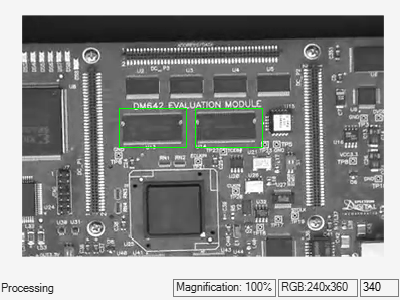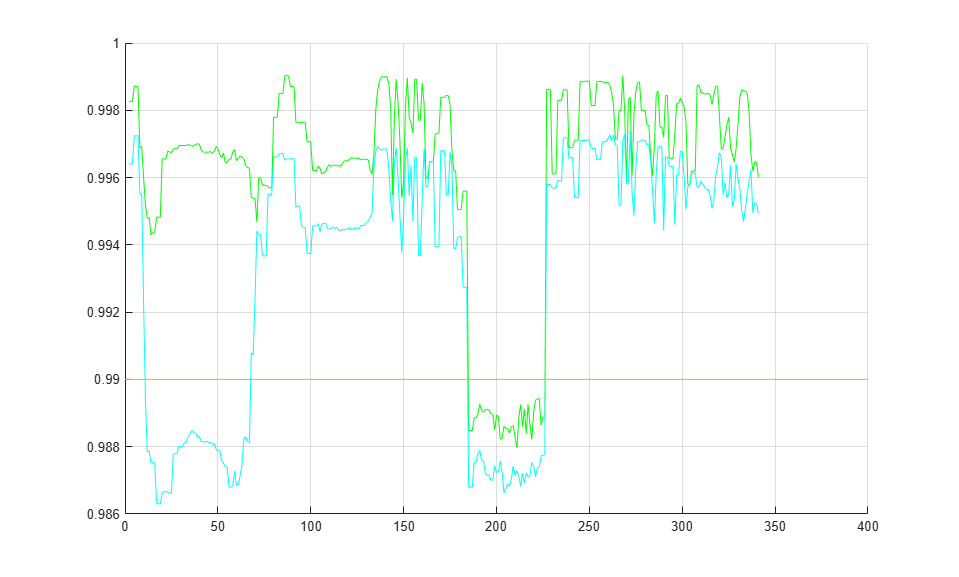# Pattern Matching

This example shows how to use the 2-D normalized cross-correlation for pattern matching and target tracking. The example uses predefined or user specified target and number of similar targets to be tracked. The normalized cross correlation plot shows that when the value exceeds the set threshold, the target is identified.

### Introduction

In this example you use normalized cross correlation to track a target pattern in a video. The pattern matching algorithm involves the following steps:

• The input video frame and the template are reduced in size to minimize the amount of computation required by the matching algorithm.

• Normalized cross correlation, in the frequency domain, is used to find a template in the video frame.

• The location of the pattern is determined by finding the maximum cross correlation value.

### Initialize Parameters and Create a Template

Initialize required variables such as the threshold value for the cross correlation and the decomposition level for Gaussian Pyramid decomposition.

```threshold = single(0.99); level = 2; ```

```hVideoSrc = VideoReader('vipboard.mp4'); ```

Specify the target image and number of similar targets to be tracked. By default, the example uses a predefined target and finds up to 2 similar patterns. You can set the variable useDefaultTarget to false to specify a new target and the number of similar targets to match.

```useDefaultTarget = true; [Img, numberOfTargets, target_image] = ... videopattern_gettemplate(useDefaultTarget); % Downsample the target image by a predefined factor. You do this % to reduce the amount of computation needed by cross correlation. target_image = single(target_image); target_dim_nopyramid = size(target_image); target_image_gp = multilevelPyramid(target_image, level); target_energy = sqrt(sum(target_image_gp(:).^2)); % Rotate the target image by 180 degrees, and perform zero padding so that % the dimensions of both the target and the input image are the same. target_image_rot = imrotate(target_image_gp, 180); [rt, ct] = size(target_image_rot); Img = single(Img); Img = multilevelPyramid(Img, level); [ri, ci]= size(Img); r_mod = 2^nextpow2(rt + ri); c_mod = 2^nextpow2(ct + ci); target_image_p = [target_image_rot zeros(rt, c_mod-ct)]; target_image_p = [target_image_p; zeros(r_mod-rt, c_mod)]; % Compute the 2-D FFT of the target image target_fft = fft2(target_image_p); % Initialize constant variables used in the processing loop. target_size = repmat(target_dim_nopyramid, [numberOfTargets, 1]); gain = 2^(level); Im_p = zeros(r_mod, c_mod, 'single'); % Used for zero padding C_ones = ones(rt, ct, 'single'); % Used to calculate mean using conv ```

Create a System object to calculate the local maximum value for the normalized cross correlation.

```hFindMax = vision.LocalMaximaFinder( ... 'Threshold', single(-1), ... 'MaximumNumLocalMaxima', numberOfTargets, ... 'NeighborhoodSize', floor(size(target_image_gp)/2)*2 - 1); ```

Create a System object to display the tracking of the pattern.

```sz = get(0,'ScreenSize'); pos = [20 sz(4)-400 400 300]; hROIPattern = vision.VideoPlayer('Name', 'Overlay the ROI on the target', ... 'Position', pos); ```

Initialize figure window for plotting the normalized cross correlation value

```hPlot = videopatternplots('setup',numberOfTargets, threshold); ```

### Search for a Template in Video

Create a processing loop to perform pattern matching on the input video. This loop uses the System objects you instantiated above. The loop is stopped when you reach the end of the input file, which is detected by the `VideoReader` object.

```while hasFrame(hVideoSrc) Im = rgb2gray(im2single(readFrame(hVideoSrc))); % Reduce the image size to speed up processing Im_gp = multilevelPyramid(Im, level); % Frequency domain convolution. Im_p(1:ri, 1:ci) = Im_gp; % Zero-pad img_fft = fft2(Im_p); corr_freq = img_fft .* target_fft; corrOutput_f = ifft2(corr_freq); corrOutput_f = corrOutput_f(rt:ri, ct:ci); % Calculate image energies and block run tiles that are size of % target template. IUT_energy = (Im_gp).^2; IUT = conv2(IUT_energy, C_ones, 'valid'); IUT = sqrt(IUT); % Calculate normalized cross correlation. norm_Corr_f = (corrOutput_f) ./ (IUT * target_energy); xyLocation = step(hFindMax, norm_Corr_f); % Calculate linear indices. linear_index = sub2ind([ri-rt, ci-ct]+1, xyLocation(:,2),... xyLocation(:,1)); norm_Corr_f_linear = norm_Corr_f(:); norm_Corr_value = norm_Corr_f_linear(linear_index); detect = (norm_Corr_value > threshold); target_roi = zeros(length(detect), 4); ul_corner = (gain.*(xyLocation(detect, :)-1))+1; target_roi(detect, :) = [ul_corner, fliplr(target_size(detect, :))]; % Draw bounding box. Imf = insertShape(Im, 'Rectangle', target_roi, 'Color', 'green'); % Plot normalized cross correlation. videopatternplots('update',hPlot,norm_Corr_value); step(hROIPattern, Imf); end snapnow % Function to compute pyramid image at a particular level. function outI = multilevelPyramid(inI, level) I = inI; outI = I; for i=1:level outI = impyramid(I, 'reduce'); I = outI; end end ```### Summary

This example shows use of Computer Vision Toolbox™ to find a user defined pattern in a video and track it. The algorithm is based on normalized frequency domain cross correlation between the target and the image under test. The video player window displays the input video with the identified target locations. Also a figure displays the normalized correlation between the target and the image which is used as a metric to match the target. As can be seen whenever the correlation value exceeds the threshold (indicated by the blue line), the target is identified in the input video and the location is marked by the green bounding box.

### Appendix

The following helper functions are used in this example.